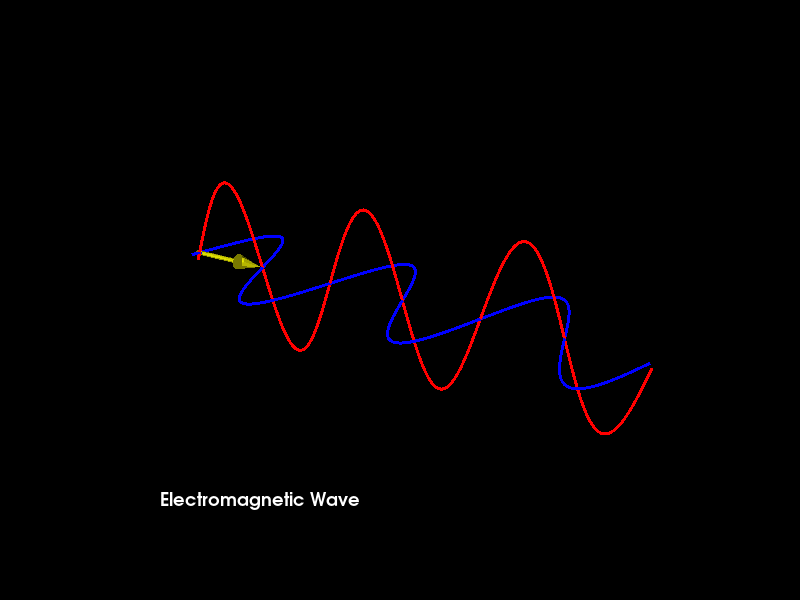# Electromagnetic Wave Propagation Animation¶

A linearly polarized sinusoidal electromagnetic wave, propagating in the direction +x through a homogeneous, isotropic, dissipationless medium, such as vacuum. The electric field (blue arrows) oscillates in the ±z-direction, and the orthogonal magnetic field (red arrows) oscillates in phase with the electric field, but in the ±y-direction.

Function of the sinusoid used in the animation = sin(k*x - w*t + d) Where, k:wavenumber, x:abscissa, w:angular frequency, t:time, d:phase angle

Importing necessary modules

```from fury import window, actor, utils, ui
import numpy as np
import itertools
```

function that updates and returns the coordinates of the waves which are changing with time

```def update_coordinates(wavenumber, ang_frq, time, phase_angle):
x = np.linspace(-3, 3, npoints)
y = np.sin(wavenumber*x - ang_frq*time + phase_angle)
z = np.array([0 for i in range(npoints)])
return x, y, z
```

Variable(s) and their description- npoints: For high quality rendering, keep the number of npoints high

but kindly note that higher values for npoints will slow down the rendering process (default = 800)

wavelength : wavelength of the wave (default = 2) wavenumber : 2*pi/wavelength time: time (default time i.e. time at beginning of the animation = 0) incre_time: value by which time is incremented for each call of

timer_callback (default = 0.1)

angular_frq: angular frequency (default = 0.1) phase_angle: phase angle (default = 0.002)

```npoints = 800
wavelength = 2
wavenumber = 2*np.pi/wavelength
time = 0
incre_time = 0.1
angular_frq = 0.1
phase_angle = 0.002
```

Creating a scene object and configuring the camera’s position

```scene = window.Scene()
scene.set_camera(position=(-6, 5, -10), focal_point=(0.0, 0.0, 0.0),
view_up=(0.0, 0.0, 0.0))
showm = window.ShowManager(scene,
size=(800, 600), reset_camera=True,
order_transparent=True)
showm.initialize()
```

Creating a yellow colored arrow to show the direction of propagation of electromagnetic wave

```centers = np.array([[3, 0, 0]])
directions = np.array([[-1, 0, 0]])
heights = np.array([6.4])
arrow_actor = actor.arrow(centers, directions, window.colors.yellow, heights,
```

Creating point actor that renders the magnetic field

```x = np.linspace(-3, 3, npoints)
y = np.sin(wavenumber*x - angular_frq*time + phase_angle)
z = np.array([0 for i in range(npoints)])

pts = np.array([(a, b, c) for (a, b, c) in zip(x, y, z)])
pts = [pts]
colors = window.colors.red
wave_actor1 = actor.line(pts, colors, linewidth=3)

vertices = utils.vertices_from_actor(wave_actor1)
vcolors = utils.colors_from_actor(wave_actor1, 'colors')
no_vertices_per_point = len(vertices)/npoints
initial_vertices = vertices.copy() - \
np.repeat(pts, no_vertices_per_point, axis=0)
```

Creating point actor that renders the electric field

```xx = np.linspace(-3, 3, npoints)
yy = np.array([0 for i in range(npoints)])
zz = np.sin(wavenumber*xx - angular_frq*time + phase_angle)

pts2 = np.array([(a, b, c) for (a, b, c) in zip(xx, yy, zz)])
pts2 = [pts2]
colors2 = window.colors.blue
wave_actor2 = actor.line(pts2, colors2, linewidth=3)

vertices2 = utils.vertices_from_actor(wave_actor2)
vcolors2 = utils.colors_from_actor(wave_actor2, 'colors')
no_vertices_per_point2 = len(vertices2)/npoints
initial_vertices2 = vertices2.copy() - \
np.repeat(pts2, no_vertices_per_point2, axis=0)
```

Initializing text box to display the title of the animation

```tb = ui.TextBlock2D(bold=True, position=(160, 90))
tb.message = "Electromagnetic Wave"
```

end is used to decide when to end the animation

```end = 300
```

Initializing counter

```counter = itertools.count()
```

Coordinates to be plotted are changed everytime timer_callback is called by using the update_coordinates function. The wave is rendered here.

```def timer_callback(_obj, _event):
global pts, pts2, time, time_incre, angular_frq, phase_angle, wavenumber
time += incre_time
cnt = next(counter)

x, y, z = update_coordinates(wavenumber, angular_frq, phase_angle, time)
pts = np.array([(a, b, c) for (a, b, c) in zip(x, y, z)])
vertices[:] = initial_vertices + \
np.repeat(pts, no_vertices_per_point, axis=0)
utils.update_actor(wave_actor1)

xx, zz, yy = update_coordinates(wavenumber, angular_frq, phase_angle, time)
pts2 = np.array([(a, b, c) for (a, b, c) in zip(xx, yy, zz)])
vertices2[:] = initial_vertices2 + \
np.repeat(pts2, no_vertices_per_point2, axis=0)
utils.update_actor(wave_actor2)

showm.render()

# to end the animation
if cnt == end:
showm.exit()
```

Run every 25 milliseconds

```showm.add_timer_callback(True, 25, timer_callback)

interactive = False
if interactive:
showm.start()
window.record(showm.scene, size=(800, 600), out_path="viz_emwave.png")
```Total running time of the script: ( 0 minutes 0.138 seconds)

Gallery generated by Sphinx-Gallery